# 23.1.13. Vector creation¶

## 23.1.13.1. Array of offset (parallel) lines¶

Creates copies of line features in a layer, by creating multiple offset versions of each feature. Each new version is incrementally offset by a specified distance.

Positive distance will offset lines to the left, and negative distances will offset them to the right.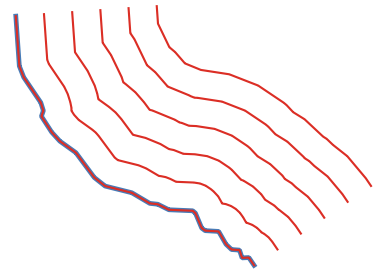Рис. 23.21 In blue the source layer, in red the offset one

См.также

### 23.1.13.1.1. Parameters¶

Label

Имя

Тип

Описание

Input layer

`INPUT`

[vector: line]

Input line vector layer to use for the offsets.

Number of features to create

`COUNT`

Default: 10

Number of offset copies to generate for each feature

Offset step distance

`OFFSET`

Default: 1.0

Distance between two consecutive offset copies

Segments

`SEGMENTS`

[number]

Default: 8

Number of line segments to use to approximate a quarter circle when creating rounded offsets

Join style

`JOIN_STYLE`

[enumeration]

Default: 0

Specify whether round, miter or beveled joins should be used when offsetting corners in a line. One of:

• 0 — Round

• 1 — Miter

• 2 — Bevel

Miter limit

`MITER_LIMIT`

[number]

Default: 2.0

Only applicable for mitered join styles, and controls the maximum distance from the offset curve to use when creating a mitered join.

Offset lines

`OUTPUT`

[vector: line]

Default: `[Create temporary layer]`

Specify the output line layer with offset features. One of:

• Create Temporary Layer (`TEMPORARY_OUTPUT`)

• Save to File…

• Save to Geopackage…

• Save to PostGIS Table…

The file encoding can also be changed here.

### 23.1.13.1.2. Outputs¶

Label

Имя

Тип

Описание

Offset lines

`OUTPUT`

[vector: line]

Output line layer with offset features. The original features are also copied.

### 23.1.13.1.3. Python code¶

Algorithm ID: `qgis:arrayoffsetlines`

```import processing
processing.run("algorithm_id", {parameter_dictionary})
```

The algorithm id is displayed when you hover over the algorithm in the Processing Toolbox. The parameter dictionary provides the parameter NAMEs and values. See Изпользование алгоритмов геообработки в консоли for details on how to run processing algorithms from the Python console.

## 23.1.13.2. Array of translated features¶

Creates copies of features in a layer by creating multiple translated versions of each. Each copy is incrementally displaced by a preset amount in the X, Y and/or Z axis.

M values present in the geometry can also be translated.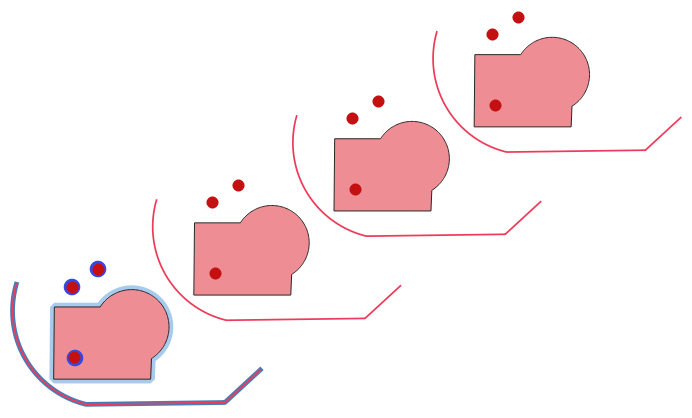Рис. 23.22 Input layers in blue tones, output layers with translated features in red tones

### 23.1.13.2.1. Parameters¶

Label

Имя

Тип

Описание

Input layer

`INPUT`

[vector: any]

Input vector layer to translate

Number of features to create

`COUNT`

Default: 10

Number of copies to generate for each feature

Step distance (x-axis)

`DELTA_X`

Default: 0.0

Displacement to apply on the X axis

Step distance (y-axis)

`DELTA_Y`

Default: 0.0

Displacement to apply on the Y axis

Step distance (z-axis)

`DELTA_Z`

Default: 0.0

Displacement to apply on the Z axis

Step distance (m values)

`DELTA_M`

Default: 0.0

Displacement to apply on M

Translated

`OUTPUT`

[same as input]

Default: `[Create temporary layer]`

Output vector layer with translated (moved) copies of the features. The original features are also copied. One of:

• Create Temporary Layer (`TEMPORARY_OUTPUT`)

• Save to File…

• Save to Geopackage…

• Save to PostGIS Table…

The file encoding can also be changed here.

### 23.1.13.2.2. Outputs¶

Label

Имя

Тип

Описание

Translated

`OUTPUT`

[same as input]

Output vector layer with translated (moved) copies of the features. The original features are also copied.

### 23.1.13.2.3. Python code¶

Algorithm ID: `qgis:arraytranslatedfeatures`

```import processing
processing.run("algorithm_id", {parameter_dictionary})
```

The algorithm id is displayed when you hover over the algorithm in the Processing Toolbox. The parameter dictionary provides the parameter NAMEs and values. See Изпользование алгоритмов геообработки в консоли for details on how to run processing algorithms from the Python console.

## 23.1.13.3. Create grid¶

Creates a vector layer with a grid covering a given extent. Grid cells can have different shapes: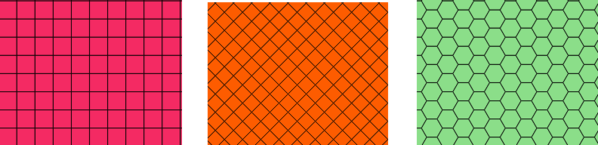Рис. 23.23 Different grid cell shapes

The size of each element in the grid is defined using a horizontal and vertical spacing.

The CRS of the output layer must be defined.

The grid extent and the spacing values must be expressed in the coordinates and units of this CRS.

Default menu: Vector ▶ Research Tools

### 23.1.13.3.1. Parameters¶

Label

Имя

Тип

Описание

Grid type

`TYPE`

[enumeration]

Default: 0

Shape of the grid. One of:

• 0 — Point

• 1 — Line

• 2 — Rectangle (polygon)

• 3 — Diamond (polygon)

• 4 — Hexagon (polygon)

Grid extent

`EXTENT`

[extent]

Extent of the grid

Horizontal spacing

`HSPACING`

[number]

Default: 1.0

Size of a grid cell on the X-axis

Vertical spacing

`VSPACING`

[number]

Default: 1.0

Size of a grid cell on the Y-axis

Horizontal overlay

`HOVERLAY`

[number]

Default: 0.0

Overlay distance between two consecutive grid cells on the X-axis

Vertical overlay

`VOVERLAY`

[number]

Default: 0.0

Overlay distance between two consecutive grid cells on the Y-axis

Grid CRS

`CRS`

[crs]

Default: Project CRS

Coordinate reference system to apply to the grid

Grid

`OUTPUT`

[vector: any]

Default: `[Create temporary layer]`

Resulting vector grid layer. One of:

• Create Temporary Layer (`TEMPORARY_OUTPUT`)

• Save to File…

• Save to Geopackage…

• Save to PostGIS Table…

The file encoding can also be changed here.

### 23.1.13.3.2. Outputs¶

Label

Имя

Тип

Описание

Grid

`OUTPUT`

[vector: any]

Resulting vector grid layer. The output geometry type (point, line or polygon) depends on the Grid type.

### 23.1.13.3.3. Python code¶

Algorithm ID: `qgis:creategrid`

```import processing
processing.run("algorithm_id", {parameter_dictionary})
```

The algorithm id is displayed when you hover over the algorithm in the Processing Toolbox. The parameter dictionary provides the parameter NAMEs and values. See Изпользование алгоритмов геообработки в консоли for details on how to run processing algorithms from the Python console.

## 23.1.13.4. Create points layer from table¶

Creates points layer from a table with columns that contain coordinates fields.

Besides X and Y coordinates you can also specify Z and M fields.

### 23.1.13.4.1. Parameters¶

Label

Имя

Тип

Описание

Input layer

`INPUT`

[vector: any]

Input vector layer or a table.

X field

`XFIELD`

[tablefield: any]

Field containing the X coordinate

Y field

`YFIELD`

[tablefield: any]

Field containing the Y coordinate

Z field

Optional

`ZFIELD`

[tablefield: any]

Field containing the Z coordinate

M field

Optional

`MFIELD`

[tablefield: any]

Field containing the M value

Target CRS

`TARGET_CRS`

[crs]

Default: `EPSG:4326`

Coordinate reference system to use for layer. The provided coordinates are assumed to be compliant.

Points from table

`OUTPUT`

[vector: point]

Default: `[Create temporary layer]`

Specify the resulting point layer. One of:

• Create Temporary Layer (`TEMPORARY_OUTPUT`)

• Save to File…

• Save to Geopackage…

• Save to PostGIS Table…

The file encoding can also be changed here.

### 23.1.13.4.2. Outputs¶

Label

Имя

Тип

Описание

Points from table

`OUTPUT`

[vector: point]

The resulting point layer

### 23.1.13.4.3. Python code¶

Algorithm ID: `qgis:createpointslayerfromtable`

```import processing
processing.run("algorithm_id", {parameter_dictionary})
```

The algorithm id is displayed when you hover over the algorithm in the Processing Toolbox. The parameter dictionary provides the parameter NAMEs and values. See Изпользование алгоритмов геообработки в консоли for details on how to run processing algorithms from the Python console.

## 23.1.13.5. Generate points (pixel centroids) along line¶

Generates a point vector layer from an input raster and line layer.

The points correspond to the pixel centroids that intersect the line layer.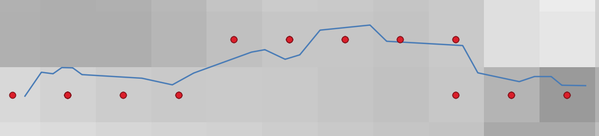Рис. 23.24 Points of the pixel centroids

### 23.1.13.5.1. Parameters¶

Label

Имя

Тип

Описание

Raster layer

`INPUT_RASTER`

[raster]

Input raster layer

Vector layer

`INPUT_VECTOR`

[vector: line]

Input line vector layer

Points along line

`OUTPUT`

[vector: point]

Default: `[Create temporary layer]`

Resulting point layer with pixel centroids. One of:

• Create Temporary Layer (`TEMPORARY_OUTPUT`)

• Save to File…

• Save to Geopackage…

• Save to PostGIS Table…

The file encoding can also be changed here.

### 23.1.13.5.2. Outputs¶

Label

Имя

Тип

Описание

Points along line

`OUTPUT`

[vector: point]

Resulting point layer with pixel centroids

### 23.1.13.5.3. Python code¶

Algorithm ID: `qgis:generatepointspixelcentroidsalongline`

```import processing
processing.run("algorithm_id", {parameter_dictionary})
```

The algorithm id is displayed when you hover over the algorithm in the Processing Toolbox. The parameter dictionary provides the parameter NAMEs and values. See Изпользование алгоритмов геообработки в консоли for details on how to run processing algorithms from the Python console.

## 23.1.13.6. Generate points (pixel centroids) inside polygon¶

Generates a point vector layer from an input raster and polygon layer.

The points correspond to the pixel centroids that intersect the polygon layer.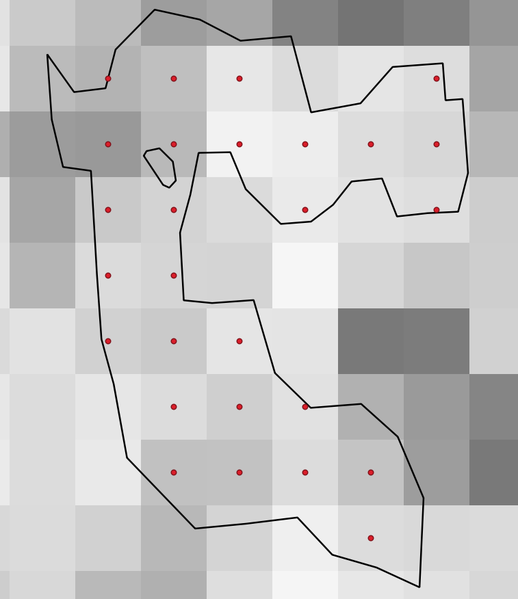Рис. 23.25 Points of the pixel centroids

### 23.1.13.6.1. Parameters¶

Label

Имя

Тип

Описание

Raster layer

`INPUT_RASTER`

[raster]

Input raster layer

Vector layer

`INPUT_VECTOR`

[vector: polygon]

Input polygon vector layer

Points inside polygons

`OUTPUT`

[vector: point]

Default: `[Create temporary layer]`

Resulting point layer of pixel centroids. One of:

• Create Temporary Layer (`TEMPORARY_OUTPUT`)

• Save to File…

• Save to Geopackage…

• Save to PostGIS Table…

The file encoding can also be changed here.

### 23.1.13.6.2. Outputs¶

Label

Имя

Тип

Описание

Points inside polygons

`OUTPUT`

[vector: point]

Resulting point layer of pixel centroids

### 23.1.13.6.3. Python code¶

Algorithm ID: `qgis:generatepointspixelcentroidsinsidepolygons`

```import processing
processing.run("algorithm_id", {parameter_dictionary})
```

The algorithm id is displayed when you hover over the algorithm in the Processing Toolbox. The parameter dictionary provides the parameter NAMEs and values. See Изпользование алгоритмов геообработки в консоли for details on how to run processing algorithms from the Python console.

## 23.1.13.7. Import geotagged photos¶

Creates a point layer corresponding to the geotagged locations from JPEG images from a source folder.

The point layer will contain a single PointZ feature per input file from which the geotags could be read. Any altitude information from the geotags will be used to set the point’s Z value.

Besides longitude and latitude also altitude, direction and timestamp information, if present in the photo, will be added to the point as attributes.

### 23.1.13.7.1. Parameters¶

Label

Имя

Тип

Описание

Input folder

`FOLDER`

[folder]

Path to the source folder containing the geotagged photos

Scan recursively

`RECURSIVE`

[boolean]

Default: False

If checked, the folder and its subfolders will be scanned

Photos

`OUTPUT`

[vector: point]

Default: `[Create temporary layer]`

Specify the point vector layer for the geotagged photos. One of:

• Create Temporary Layer (`TEMPORARY_OUTPUT`)

• Save to File…

• Save to Geopackage…

• Save to PostGIS Table…

The file encoding can also be changed here.

Invalid photos table

Optional

`INVALID`

[table]

Default: `[Skip output]`

Specify the table of unreadable or non-geotagged photos. One of:

• Skip Output

• Create Temporary Layer (`TEMPORARY_OUTPUT`)

• Save to File…

• Save to Geopackage…

• Save to PostGIS Table…

The file encoding can also be changed here.

### 23.1.13.7.2. Outputs¶

Label

Имя

Тип

Описание

Photos

`OUTPUT`

[vector: point]

Point vector layer with geotagged photos. The form of the layer is automatically filled with paths and photo previews settings.

Invalid photos table

Optional

`INVALID`

[table]

Table of unreadable or non-geotagged photos can also be created.

### 23.1.13.7.3. Python code¶

Algorithm ID: `qgis:importphotos`

```import processing
processing.run("algorithm_id", {parameter_dictionary})
```

The algorithm id is displayed when you hover over the algorithm in the Processing Toolbox. The parameter dictionary provides the parameter NAMEs and values. See Изпользование алгоритмов геообработки в консоли for details on how to run processing algorithms from the Python console.

## 23.1.13.8. Points to path¶

Converts a point layer to a line layer, by joining points in an order defined by a field in the input point layer (if the order field is a date/time field, the format must be specified).

Points can be grouped by a field to distinguish line features.

In addition to the line vector layer, a text file is output that describes the resulting line as a start point and a sequence of bearings / directions (relative to azimuth) and distances.

### 23.1.13.8.1. Parameters¶

Label

Имя

Тип

Описание

Input point layer

`INPUT`

[vector: point]

Input point vector layer

Order field

`ORDER_FIELD`

[tablefield: any]

Field containing the order to connect the points in the path

Group field

Optional

`GROUP_FIELD`

[tablefield: any]

Point features of the same value in the field will be grouped in the same line. If not set, a single path is drawn with all the input points.

Date format (if order field is DateTime)

Optional

`DATE_FORMAT`

[string]

The format to use for the `Order field` parameter. Specify this only if the `Order field` is of type Date/Time.

Paths

`OUTPUT`

[vector: line]

Default: `[Create temporary layer]`

Specify the line vector layer of the path. One of:

• Create Temporary Layer (`TEMPORARY_OUTPUT`)

• Save to File…

• Save to Geopackage…

• Save to PostGIS Table…

The file encoding can also be changed here.

Directory for text output

`OUTPUT_TEXT_DIR`

[folder]

Default: `[Skip output]`

Specify the directory that will contain the description files of points and paths. One of:

• Skip Output

• Save to a Temporary Directory

• Save to Directory…

The file encoding can also be changed here.

### 23.1.13.8.2. Outputs¶

Label

Имя

Тип

Описание

Paths

`OUTPUT`

[vector: line]

Line vector layer of the path

Directory for text output

`OUTPUT`

[folder]

Directory containing description files of points and paths

### 23.1.13.8.3. Python code¶

Algorithm ID: `qgis:pointstopath`

```import processing
processing.run("algorithm_id", {parameter_dictionary})
```

The algorithm id is displayed when you hover over the algorithm in the Processing Toolbox. The parameter dictionary provides the parameter NAMEs and values. See Изпользование алгоритмов геообработки в консоли for details on how to run processing algorithms from the Python console.

## 23.1.13.9. Random points along line¶

Creates a new point layer, with points placed in the lines of another layer.

For each line in the input layer, a given number of points is added to the resulting layer. A minimum distance can be specified, to avoid points being too close to each other.

### 23.1.13.9.1. Parameters¶

Label

Имя

Тип

Описание

Input point layer

`INPUT`

[vector: line]

Input line vector layer

Number of points

`POINTS_NUMBER`

[number]

Default: 1

Number of points to create

Minimum distance between points

`MIN_DISTANCE`

[number]

Default: 0.0

The minimum distance between points

Random points

`OUTPUT`

[vector: point]

Default: `[Create temporary layer]`

The output random points. One of:

• Create Temporary Layer (`TEMPORARY_OUTPUT`)

• Save to File…

• Save to Geopackage…

• Save to PostGIS Table…

The file encoding can also be changed here.

### 23.1.13.9.2. Outputs¶

Label

Имя

Тип

Описание

Random points

`OUTPUT`

[vector: point]

The output random points layer.

### 23.1.13.9.3. Python code¶

Algorithm ID: `qgis:qgisrandompointsalongline`

```import processing
processing.run("algorithm_id", {parameter_dictionary})
```

The algorithm id is displayed when you hover over the algorithm in the Processing Toolbox. The parameter dictionary provides the parameter NAMEs and values. See Изпользование алгоритмов геообработки в консоли for details on how to run processing algorithms from the Python console.

## 23.1.13.10. Random points in extent¶

Creates a new point layer with a given number of random points, all of them within a given extent.

A minimum distance can be specified, to avoid points being too close to each other.

Default menu: Vector ▶ Research Tools

### 23.1.13.10.1. Parameters¶

Label

Имя

Тип

Описание

Input extent

`EXTENT`

[extent]

Map extent for the random points

Number of points

`POINTS_NUMBER`

[number]

Default: 1

Number of point to create

Minimum distance between points

`MIN_DISTANCE`

[number]

Default: 0.0

The minimum distance between points

Target CRS

`TARGET_CRS`

[crs]

Default: Project CRS

CRS of the random points layer

Random points

`OUTPUT`

[vector: point]

Default: `[Create temporary layer]`

The output random points. One of:

• Create Temporary Layer (`TEMPORARY_OUTPUT`)

• Save to File…

• Save to Geopackage…

• Save to PostGIS Table…

The file encoding can also be changed here.

### 23.1.13.10.2. Outputs¶

Label

Имя

Тип

Описание

Random points

`OUTPUT`

[vector: point]

The output random points layer.

### 23.1.13.10.3. Python code¶

Algorithm ID: `qgis:randompointsinextent`

```import processing
processing.run("algorithm_id", {parameter_dictionary})
```

The algorithm id is displayed when you hover over the algorithm in the Processing Toolbox. The parameter dictionary provides the parameter NAMEs and values. See Изпользование алгоритмов геообработки в консоли for details on how to run processing algorithms from the Python console.

## 23.1.13.11. Random points in layer bounds¶

Creates a new point layer with a given number of random points, all of them within the extent of a given layer.

A minimum distance can be specified, to avoid points being too close to each other.

Default menu: Vector ▶ Research Tools

### 23.1.13.11.1. Parameters¶

Label

Имя

Тип

Описание

Input layer

`INPUT`

[vector: polygon]

Input polygon layer defining the area

Number of points

`POINTS_NUMBER`

[number]

Default: 1

Number of points to create

Minimum distance between points

`MIN_DISTANCE`

[number]

Default: 0.0

The minimum distance between points

Random points

`OUTPUT`

[vector: point]

Default: `[Create temporary layer]`

The output random points. One of:

• Create Temporary Layer (`TEMPORARY_OUTPUT`)

• Save to File…

• Save to Geopackage…

• Save to PostGIS Table…

The file encoding can also be changed here.

### 23.1.13.11.2. Outputs¶

Label

Имя

Тип

Описание

Random points

`OUTPUT`

[vector: point]

The output random points layer.

### 23.1.13.11.3. Python code¶

Algorithm ID: `qgis:randompointsinlayerbounds`

```import processing
processing.run("algorithm_id", {parameter_dictionary})
```

The algorithm id is displayed when you hover over the algorithm in the Processing Toolbox. The parameter dictionary provides the parameter NAMEs and values. See Изпользование алгоритмов геообработки в консоли for details on how to run processing algorithms from the Python console.

## 23.1.13.12. Random points inside polygons¶

Creates a new point layer with a given number of random points inside each polygon of the input polygon layer.

Two sampling strategies are available:

• Points count: number of points for each feature

• Points density: density of points for each feature

A minimum distance can be specified, to avoid points being too close to each other.

Default menu: Vector ▶ Research Tools

### 23.1.13.12.1. Parameters¶

Label

Имя

Тип

Описание

Input layer

`INPUT`

[vector: polygon]

Input polygon vector layer

Sampling strategy

`STRATEGY`

[enumeration]

Default: 0

Sampling strategy to use. One of:

• 0 — Points count: number of points for each feature

• 1 — Points density: density of points for each feature

Point count or density

`VALUE`

Default: 1.0

The number or density of points, depending on the chosen Sampling strategy.

Minimum distance between points

`MIN_DISTANCE`

[number]

Default: 0.0

The minimum distance between points

Random points

`OUTPUT`

[vector: point]

Default: `[Create temporary layer]`

The output random points. One of:

• Create Temporary Layer (`TEMPORARY_OUTPUT`)

• Save to File…

• Save to Geopackage…

• Save to PostGIS Table…

The file encoding can also be changed here.

### 23.1.13.12.2. Outputs¶

Label

Имя

Тип

Описание

Random points

`OUTPUT`

[vector: point]

The output random points layer.

### 23.1.13.12.3. Python code¶

Algorithm ID: `qgis:randompointsinsidepolygons`

```import processing
processing.run("algorithm_id", {parameter_dictionary})
```

The algorithm id is displayed when you hover over the algorithm in the Processing Toolbox. The parameter dictionary provides the parameter NAMEs and values. See Изпользование алгоритмов геообработки в консоли for details on how to run processing algorithms from the Python console.

## 23.1.13.13. Raster pixels to points¶

Creates a vector layer of points corresponding to each pixel in a raster layer.

Converts a raster layer to a vector layer, by creating point features for each individual pixel’s center in the raster layer. Any nodata pixels are skipped in the output.

### 23.1.13.13.1. Parameters¶

Label

Имя

Тип

Описание

Raster layer

`INPUT_RASTER`

[raster]

Input raster layer

Band number

`RASTER_BAND`

[raster band]

Raster band to extract data from

Field name

`FIELD_NAME`

[string]

Default: „VALUE“

Name of the field to store the raster band value

Vector points

`OUTPUT`

[vector: point]

Default: `[Create temporary layer]`

Specify the resulting point layer of pixels centroids. One of:

• Create Temporary Layer (`TEMPORARY_OUTPUT`)

• Save to File…

• Save to Geopackage…

• Save to PostGIS Table…

The file encoding can also be changed here.

### 23.1.13.13.2. Outputs¶

Label

Имя

Тип

Описание

Vector points

`OUTPUT`

[vector: point]

Resulting point layer with pixels centroids

### 23.1.13.13.3. Python code¶

Algorithm ID: `qgis:pixelstopoints`

```import processing
processing.run("algorithm_id", {parameter_dictionary})
```

The algorithm id is displayed when you hover over the algorithm in the Processing Toolbox. The parameter dictionary provides the parameter NAMEs and values. See Изпользование алгоритмов геообработки в консоли for details on how to run processing algorithms from the Python console.

## 23.1.13.14. Raster pixels to polygons¶

Creates a vector layer of polygons corresponding to each pixel in a raster layer.

Converts a raster layer to a vector layer, by creating polygon features for each individual pixel’s extent in the raster layer. Any nodata pixels are skipped in the output.

### 23.1.13.14.1. Parameters¶

Label

Имя

Тип

Описание

Raster layer

`INPUT_RASTER`

[raster]

Input raster layer

Band number

`RASTER_BAND`

[raster band]

Raster band to extract data from

Field name

`FIELD_NAME`

[string]

Default: „VALUE“

Name of the field to store the raster band value

Vector polygons

`OUTPUT`

[vector: polygon]

Default: `[Create temporary layer]`

Specify the resulting polygon layer of pixel extents. One of:

• Create Temporary Layer (`TEMPORARY_OUTPUT`)

• Save to File…

• Save to Geopackage…

• Save to PostGIS Table…

The file encoding can also be changed here.

### 23.1.13.14.2. Outputs¶

Label

Имя

Тип

Описание

Vector polygons

`OUTPUT`

[vector: polygon]

Resulting polygon layer of pixel extents

### 23.1.13.14.3. Python code¶

Algorithm ID: `qgis:pixelstopolygons`

```import processing
processing.run("algorithm_id", {parameter_dictionary})
```

The algorithm id is displayed when you hover over the algorithm in the Processing Toolbox. The parameter dictionary provides the parameter NAMEs and values. See Изпользование алгоритмов геообработки в консоли for details on how to run processing algorithms from the Python console.

## 23.1.13.15. Regular points¶

Creates a new point layer with its points placed in a regular grid within a given extent.

The grid is specified either by the spacing between the points (same spacing for all dimensions) or by the number of points to generate. In the latter case, the spacing will be determined from the extent. In order to generate a full rectangular grid, at least the number of points specified by the user is generated for the latter case.

Random offsets to the point spacing can be applied, resulting in a non-regular point pattern.

Default menu: Vector ▶ Research Tools

### 23.1.13.15.1. Parameters¶

Label

Имя

Тип

Описание

Input extent (xmin, xmax, ymin, ymax)

`EXTENT`

[extent]

Map extent for the random points

Point spacing/count

`SPACING`

[number]

Default: 100

Spacing between the points, or the number of points, depending on whether `Use point spacing` is checked or not.

Initial inset from corner (LH side)

`INSET`

[number]

Default: 0.0

Offsets the points relative to the upper left corner. The value is used for both the X and Y axis.

Apply random offset to point spacing

`RANDOMIZE`

[boolean]

Default: False

If checked the points will have a random spacing

Use point spacing

`IS_SPACING`

[boolean]

Default: True

If unchecked the point spacing is not taken into account

Output layer CRS

`CRS`

[crs]

Default: Project CRS

CRS of the random points layer

Regular points

`OUTPUT`

[vector: point]

Default: `[Create temporary layer]`

Specify the output regular point layer. One of:

• Create Temporary Layer (`TEMPORARY_OUTPUT`)

• Save to File…

• Save to Geopackage…

• Save to PostGIS Table…

The file encoding can also be changed here.

### 23.1.13.15.2. Outputs¶

Label

Имя

Тип

Описание

Regular points

`OUTPUT`

[vector: point]

The output regular point layer.

### 23.1.13.15.3. Python code¶

Algorithm ID: `qgis:regularpoints`

```import processing
processing.run("algorithm_id", {parameter_dictionary})
```

The algorithm id is displayed when you hover over the algorithm in the Processing Toolbox. The parameter dictionary provides the parameter NAMEs and values. See Изпользование алгоритмов геообработки в консоли for details on how to run processing algorithms from the Python console.